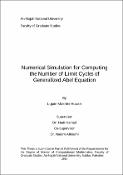## Numerical Simulation for Computing the Number of Limit Cycles of Generalized Abel Equation

 dc.contributor.author حواري, لجين مخلص dc.date.accessioned 2021-07-06T06:23:27Z dc.date.available 2021-07-06T06:23:27Z dc.date.issued 2021-02-02 dc.description.abstract Limit cycles (isolated periodic solutions) describe the phenomenon of oscillation that are considered and studied in different research fields such as physics, medicine, chemistry, populations…etc. In nature, some of biological and physical processes are represented by stable limit cycles. The interest point of this problem comes from the study of number of isolated closed orbits of a planar polynomial vector field, which is a part of Hilbert`s Sixteenth Problem; this problem has been one of the major problems in the qualitative theory of ordinary differential equations. Hilbert's problem is interested in the number of limit cycles for the planar polynomial differential system. In this work, both limit cycles in xy-plane and the stability types of limit cycles were exhibited, also direction field was considered, which describes graphically the behavior for the solution of the differential equation. Theorems related to the existence and non-existence of limit cycles were discussed. Moreover, a common nonlinear ordinary differential equation, named Abel differential equation, was discussed. Also this work presented limit cycles of first order polynomial differential equation where the coefficients are periodic, and presented some results concerned to the maximum number of limit cycles for polynomial differential equations, and work on proving these results numerically. Furthermore, limit cycles of planar differential system (planar vector field) were presented. Also the Poincaré map, multiplicity of limit cycles for planar differential system, and the multi-parameter of differential system were exhibited. Finally, the number of non-contractible limit cycles of a system in the cylinder was presented with numerical example. The most challenging problem in this work is to obtain numerical examples such that they contain more than one limit cycle by defining suitable interval, coefficients and initial conditions that satisfy relevant theorems and corollaries. While the second problem was to explore examples of limit cycles which have multiplicity greater than one. en_US dc.identifier.uri https://hdl.handle.net/20.500.11888/15901 dc.language.iso en_US en_US dc.publisher An-Najah National University en_US dc.title Numerical Simulation for Computing the Number of Limit Cycles of Generalized Abel Equation en_US dc.title.alternative محاكاة رقمية لحساب عدد دورات الحد لمعادلة أبل العامة en_US dc.type Thesis en_US
##### Original bundle
Now showing 1 - 1 of 1Name:
thesis25--2021.pdf
Size:
5.83 MB
Format:
Description:
Full Text